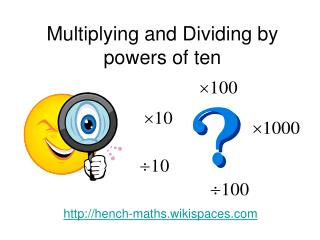DownloadDownload PresentationMultiplying and Dividing by powers of ten

# Multiplying and Dividing by powers of ten

Télécharger la présentation## Multiplying and Dividing by powers of ten

- - - - - - - - - - - - - - - - - - - - - - - - - - - E N D - - - - - - - - - - - - - - - - - - - - - - - - - - -
##### Presentation Transcript

1. Multiplying and Dividing by powers of ten http://hench-maths.wikispaces.com

2. 5 3 7 4 8 2 What are the PLACE VALUES of the digits in this number?

3. 5 3 7 4 8 2 So that means 500 + 30 + 7 + + +

4. 5 3 7 4 8 2 The decimal point just separates the whole numbers from the decimal fractions

5. 5 3 7 4 8 2 What happens when I multiply this number by 10 ?

6. 5 3 7 4 8 2 Each digits value will become 10 times bigger so the digits move LEFT into the next column

7. Multiplying by 10 537.482 x 10 = 5374.82 It LOOKS like the decimal point moves one place to the right. (and that is how many teachers ask you to think of it)

8. Multiplying by 10 Moving the digits one place to the LEFT looks the same as moving the decimal point one place to the RIGHT. 5 3 7 4 8 2 5 3 7 4 8 2

9. More Multiplying and Dividing

10. I hope I haven’t LEFT you confused but I thought we should get this RIGHT Thanks for watching.